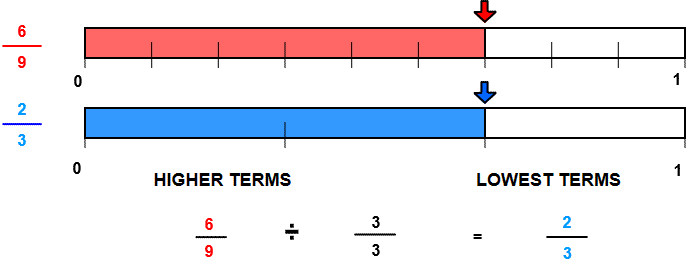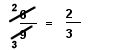# Rename to lowest terms with number lines

## Rename fractions to lowest terms is pictured with number line models.

RENAME to LOWEST TERMS
CORRECT:
ATTEMPTS:
SCORE:
PERCENT
NUMERATOR
DENOMINATOR
HIGHER TERMS
LOWEST TERMS

# RENAME TO LOWEST TERMS WITH LINES INSTRUCTIONS

Follow the directions in the dialog box after pressing the <START> button. The <EXPLAIN> button may be pressed to see how to do the example.

Rename to Lowest Terms uses number lines to demonstrate how a fraction can be renamed to lowest terms.

The following equivalent fractions were made by Rename to Lowest Terms with Lines Designer:When the program starts, you will see a fraction in higher terms. In the above illustration, the fraction 69 is pointed to by the red arrow.

You are to write the fraction in lowest terms. To do so, think of the largest number that will divide evenly into both the numerator and the denominator. In the example above, the first fraction has a numerator of 6 and a denominator of 9. The largest number that divides evenly into both 6 and 9 is 3. Three (3) is the greatest common factor of 6 and 9.

The numerator of the second fraction is 2 because 6 divided by 3 is 2.
The denominator of the second fraction is 3 because 9 divided by 3 is 3.

You can see by the illustration that you are actually dividing by 33, a form of one(1).

After an equivalent fraction in lowest terms is entered,  the number line for the second fraction will appear. The arrow along the second number line is the same distance as the arrow along the first number line. Although there are less parts in the second number line, the parts are larger, making the two fractions equivalent.

It is important to know that both 69 and 23 are equivalent fractions. You are not "reducing" the fraction 69 by renaming in lowest terms. Some websites and even some textbooks use the term "reduce" but this can cause confusion to many learners.

When renaming 69 as 23 you are "writing in lowest terms" or you are " simplifying" 69.

The fraction on the right is in lowest terms because there is no number larger than 1 that can divide evenly into both the numerator and the denominator.

For more instruction on renaming fractions to lowest terms go to How To Rename to Lower Terms.

After you enter first example you may press the <REPORT> button. This report will give same results as on the dialog box. The report will ask for your name but you may submit a code for your name. The report may be printed or e-mailed.

Another way to write the example is to divide the numerator by the greatest common factor and write the answer over the numerator. Then draw a line through the numerator. Do the same to the denominator, but write the answer under the denominator. This is known as canceling.

See How to Cancel, a short animation on canceling.

The example would look like this if you cancel: